# Petrie polygon

Various visualizations of the icosahedron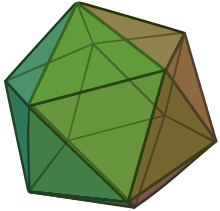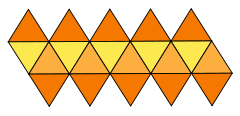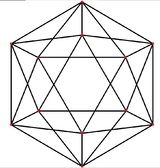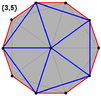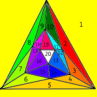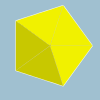In geometry, a Petrie polygon for a regular polytope of n dimensions is a skew polygon such that every (n-1) consecutive sides (but no n) belong to one of the facets. The Petrie polygon of a regular polygon is the regular polygon itself; that of a regular polyhedron is a skew polygon such that every two consecutive sides (but no three) belong to one of the faces.

For every regular polytope there exists an orthogonal projection onto a plane such that one Petrie polygon becomes a regular polygon with the remainder of the projection interior to it. The plane in question is the Coxeter plane of the symmetry group of the polygon, and the number of sides, h, is Coxeter number of the Coxeter group. These polygons and projected graphs are useful in visualizing symmetric structure of the higher-dimensional regular polytopes.

## History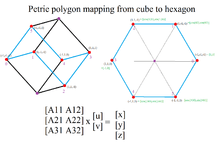The Petrie polygon for a cube is a skew hexagon passing through 6 of 8 vertices. The skew Petrie polygon can be seen as a regular planar polygon by a specific orthogonal projection.

John Flinders Petrie (1907–1972) was the only son of Egyptologist Flinders Petrie. He was born in 1907 and as a schoolboy showed remarkable promise of mathematical ability. In periods of intense concentration he could answer questions about complicated four-dimensional objects by visualizing them.

He first noted the importance of the regular skew polygons which appear on the surface of regular polyhedra and higher polytopes. Coxeter explained in 1937 how he and Petrie began to expand the classical subject of regular polyhedra:

One day in 1926, J. F. Petrie told me with much excitement that he had discovered two new regular polyhedral; infinite but free of false vertices. When my incredulity had begun to subside, he described them to me: one consisting of squares, six at each vertex, and one consisting of hexagons, four at each vertex.

In 1938 Petrie collaborated with Coxeter, Patrick du Val, and H.T. Flather to produce The Fifty-Nine Icosahedra for publication. Realizing the geometric facility of the skew polygons used by Petrie, Coxeter named them after his friend when he wrote Regular Polytopes.

In 1972, a few months after his retirement, Petrie was killed by a car while attempting to cross a motorway near his home in Surrey.

The idea of Petrie polygons was later extended to semiregular polytopes.

## The Petrie polygons of the regular polyhedra

The Petrie polygon of the regular polyhedron {p, q} has h sides, where

h+2=24/(10-p-q).

The regular duals, {p,q} and {q,p}, are contained within the same projected Petrie polygon.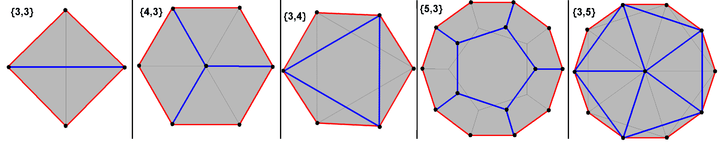tetrahedron cube octahedron dodecahedron icosahedronedge-centered vertex-centered face-centered face-centered vertex-centered 4 sides 6 sides 6 sides 10 sides 10 sides V:(4,0) V:(6,2) V:(6,0) V:(10,10,0) V:(10,2) The Petrie polygons are the exterior of these orthogonal projections. Blue show "front" edges, while black lines show back edges. The concentric rings of vertices are counted starting from the outside working inwards with a notation: V:(a, b, ...), ending in zero if there are no central vertices.

Infinite regular skew polygons (apeirogon) can also be defined as being the Petrie polygons of the regular tilings, having angles of 90, 120, and 60 degrees of their square, hexagon and triangular faces respectively.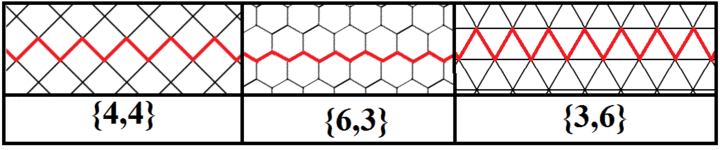Infinite regular skew polygons also exist as Petrie polygons of the regular hyperbolic tilings, like the order-7 triangular tiling, {3,7}: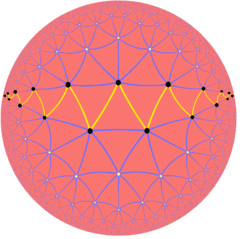## The Petrie polygon of regular polychora (4-polytopes)

The Petrie polygon for the regular polychora {p, q ,r} can also be determined.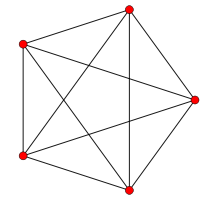{3,3,3}5-cell5 sidesV:(5,0)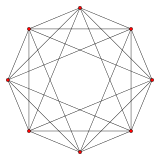{3,3,4}16-cell8 sidesV:(8,0)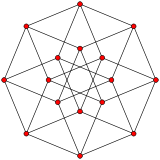{4,3,3}tesseract8 sidesV:(8,8,0)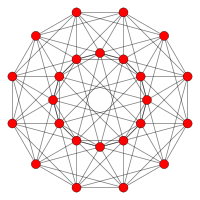{3,4,3}24-cell12 sidesV:(12,6,6,0)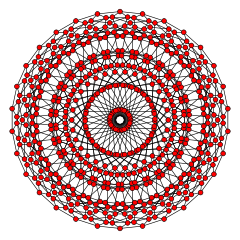{5,3,3}120-cell30 sidesV:((30,60)3,603,30,60,0)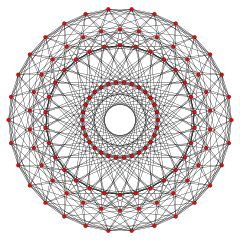{3,3,5}600-cell30 sidesV:(30,30,30,30,0)

## The Petrie polygon projections of regular and uniform polytopes

The Petrie polygon projections are most useful for visualization of polytopes of dimension four and higher. This table represents Petrie polygon projections of 3 regular families (simplex, hypercube, orthoplex), and the exceptional Lie group En which generate semiregular and uniform polytopes for dimensions 4 to 8.

## Petrie dual

A Petrie dual or Petrial of a regular polyhedron is a regular map whose vertices and edges correspond to the vertices and edges of the original polyhedron, and whose faces are the set of petrie polygons, and is expressed by a π superscript operator to a regular polytope. Each edge is shared by 2 petrie polygon faces.

The petrial tetrahedron, {3,3}π, has 4 vertices, 6 edges, and 3 skew square faces. With an Euler characteristic, χ, of 1, it is topologically identical to the hemi-cube, {4,3}/2.

The petrial cube, {4,3}π, has 8 vertices, 12 edges, and 4 skew hexagons, colored red, green, blue and orange here. With an Euler characteristic of 0, it can also be seen in the four hexagonal faces of the toroidal hexagonal tiling {6,3}(2,0).

A petrial octahedron, {3,4}π, has 6 vertices, 12 edges, and 4 skew hexagon faces. It has an Euler characteristic of -2, and has a mapping to the hyperbolic order-4 hexagonal tiling, as {6,4}3.

A petrial dodecahedron, {5,3}π, has 20 vertices, 30 edges, and 6 skew decagonal faces, and Euler characteristic of -4, related to the hyperbolic tiling {10,3}5.

A petrial icosahedron, {3,5}π, has 12 vertices, 30 edges, and 6 skew decagonal faces, and Euler characteristic of -12, related to the hyperbolic tiling {10,5}3.

Regular petrials
Petrial tetrahedron
{3,3}π = {4,3}3 = {4,3}/2
Petrial cube
{4,3}π = {6,3}3 = {6,3}(2,0)
Petrial octahedron
{3,4}π = {6,4}3
Petrial dodecahedron
{5,3}π = {10,3}5.
Petrial icosahedron
{3,5}π = {10,5}3.
3 skew squares 4 skew hexagons 6 skew decagons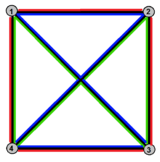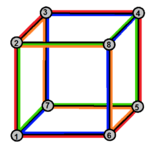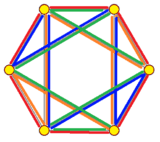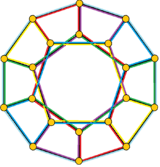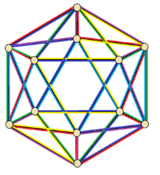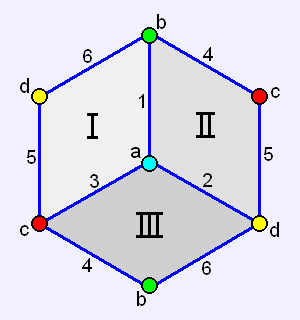{4,3}3 = {4,3}/2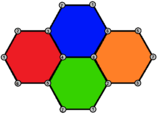{6,3}3 = {6,3}(2,0)

## Notes

1. Kaleidoscopes: Selected Writings of H. S. M. Coxeter, edited by F. Arthur Sherk, Peter McMullen, Anthony C. Thompson, Asia Ivic Weiss, Wiley-Interscience Publication, 1995, ISBN 978-0-471-01003-6 (Definition: paper 13, Discrete groups generated by reflections, 1933, p. 161)
2. H.S.M. Coxeter (1937) "Regular skew polyhedral in three and four dimensions and their topological analogues", Proceedings of the London Mathematical Society (2) 43: 33 to 62
3. H. S. M. Coxeter, Patrick du Val, H.T. Flather, J.F. Petrie (1938) The Fifty-nine Icosahedra, University of Toronto studies, mathematical series 6: 1–26
4. H.S.M. Coxeter (1973) Regular Polytopes, 3rd edition, page 32
5. http://cms.math.ca/openaccess/cjm/v10/cjm1958v10.0220-0221.pdf
6. Abstract regular polytopes, 7B operations on regular polytopes, pp.192-200, Petrie operation, π
7. http://www.weddslist.com/rmdb/pages/glossary.php#petrial
9. Coxeter–Petrie Complexes of Regular Maps

## References

• Coxeter, H. S. M. (1947, 63, 73) Regular Polytopes, 3rd ed. New York: Dover, 1973. (sec 2.6 Petrie Polygons pp. 2425, and Chapter 12, pp. 213–235, The generalized Petrie polygon )
• Coxeter, H.S.M. (1974) Regular complex polytopes. Section 4.3 Flags and Orthoschemes, Section 11.3 Petrie polygons
• Ball, W. W. R. and H. S. M. Coxeter (1987) Mathematical Recreations and Essays, 13th ed. New York: Dover. (p. 135)
• Coxeter, H. S. M. (1999) The Beauty of Geometry: Twelve Essays, Dover Publications LCCN 99-35678
• Peter McMullen, Egon Schulte (2002) Abstract Regular Polytopes, Cambridge University Press. ISBN 0-521-81496-0
• Steinberg, Robert,ON THE NUMBER OF SIDES OF A PETRIE POLYGON

Fundamental convex regular and uniform polytopes in dimensions 2–10
Family An Bn I2(p) / Dn E6 / E7 / E8 / E9 / E10 / F4 / G2 Hn
Regular polygon Triangle Square p-gon Hexagon Pentagon
Uniform polyhedron Tetrahedron OctahedronCube Demicube DodecahedronIcosahedron
Uniform 4-polytope 5-cell 16-cellTesseract Demitesseract 24-cell 120-cell600-cell
Uniform 5-polytope 5-simplex 5-orthoplex5-cube 5-demicube
Uniform 6-polytope 6-simplex 6-orthoplex6-cube 6-demicube 122221
Uniform 7-polytope 7-simplex 7-orthoplex7-cube 7-demicube 132231321
Uniform 8-polytope 8-simplex 8-orthoplex8-cube 8-demicube 142241421
Uniform 9-polytope 9-simplex 9-orthoplex9-cube 9-demicube
Uniform 10-polytope 10-simplex 10-orthoplex10-cube 10-demicube
Uniform n-polytope n-simplex n-orthoplexn-cube n-demicube 1k22k1k21 n-pentagonal polytope
Topics: Polytope familiesRegular polytopeList of regular polytopes and compounds